# NCERT solutions for Class 10 Maths chapter 12 - Areas Related to Circles [Latest edition]

#### Chapters## Solutions for Chapter 12: Areas Related to Circles

Below listed, you can find solutions for Chapter 12 of CBSE NCERT for Class 10 Maths.

Exercise 12.1Exercise 12.2Exercise 12.3
Exercise 12.1 [Pages 225 - 226]

### NCERT solutions for Class 10 Maths Chapter 12 Areas Related to Circles Exercise 12.1 [Pages 225 - 226]

Exercise 12.1 | Q 1 | Page 225

The radii of two circles are 19 cm and 9 cm respectively. Find the radius of the circle which has circumference equal to the sum of the circumferences of the two circles.

Exercise 12.1 | Q 2 | Page 225

The radii of two circles are 8 cm and 6 cm respectively. Find the radius of the circle having area equal to the sum of the areas of the two circles.

Exercise 12.1 | Q 3 | Page 225

Given figure depicts an archery target marked with its five scoring areas from the centre outwards as Gold, Red, Blue, Black and White. The diameter of the region representing Gold score is 21 cm and each of the other bands is 10.5 cm wide. Find the area of each of the five scoring regions. [use π = 22/7]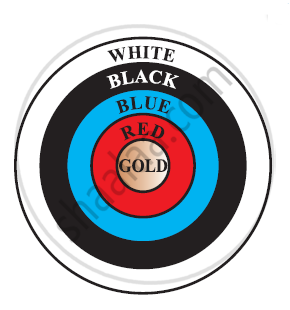Exercise 12.1 | Q 4 | Page 226

The wheels of a car are of diameter 80 cm each. How many complete revolutions does each wheel make in 10 minutes when the car is traveling at a speed of 66 km per hour? [Use Π = 22/7]

Exercise 12.1 | Q 5 | Page 226

Tick the correct answer in the following and justify your choice: If the perimeter and the area of a circle are numerically equal, then the radius of the circle is:

• 2 units

• π units

• 4 units

• 2π units

• 7 units

Exercise 12.2 [Pages 230 - 231]

### NCERT solutions for Class 10 Maths Chapter 12 Areas Related to Circles Exercise 12.2 [Pages 230 - 231]

Exercise 12.2 | Q 1 | Page 230

Find the area of a sector of a circle with radius 6 cm if angle of the sector is 60° [Use Π = 22/7]

Exercise 12.2 | Q 2 | Page 230

Find the area of a quadrant of a circle whose circumference is 22 cm. [Use pi = 22/7]

Exercise 12.2 | Q 3 | Page 230

The length of the minute hand of a clock is 14 cm. Find the area swept by the minute hand in 5 minutes.  [Use Π = 22/7]

Exercise 12.2 | Q 4 | Page 230

A chord of a circle of radius 10 cm subtends a right angle at the centre. Find the area of the corresponding:

(i) Minor segment

(ii) Major sector

[Use π = 3.14]

Exercise 12.2 | Q 5 | Page 230

In a circle of radius 21 cm, an arc subtends an angle of 60° at the centre. Find:

(i) The length of the arc

(ii) Area of the sector formed by the arc

(iii) Area of the segment forced by the corresponding chord

[use Π = 22/7]

Exercise 12.2 | Q 6 | Page 230

A chord of a circle of radius 15 cm subtends an angle of 60° at the centre. Find the areas of the corresponding minor and major segments of the circle. [Use π = 3.14 and sqrt3 = 1.73]

Exercise 12.2 | Q 7 | Page 230

A chord of a circle of radius 12 cm subtends an angle of 120° at the centre. Find the area of the corresponding segment of the circle. [Use π = 3.14 and sqrt3 = 1.73 ]

Exercise 12.2 | Q 8 | Page 230

A horse is tied to a peg at one corner of a square shaped grass field of side 15 m by means of a 5 m long rope (see the given figure). Find

(i) The area of that part of the field in which the horse can graze.

(ii) The increase in the grazing area of the rope were 10 m long instead of 5 m.

[Use π = 3.14]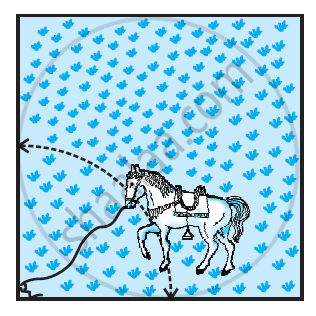Exercise 12.2 | Q 9 | Page 230

A brooch is made with silver wire in the form of a circle with diameter 35 mm. The wire is also used in making 5 diameters which divide the circle into 10 equal sectors as shown in figure. Find.

(i) The total length of the silver wire required.

(ii) The area of each sector of the brooch

[Use Π = 22/7]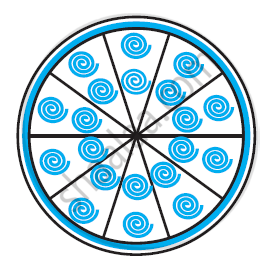Exercise 12.2 | Q 10 | Page 231

An umbrella has 8 ribs which are equally spaced. Assuming umbrella to be a flat circle of radius 45 cm. Find the area between the two consecutive ribs of the umbrella.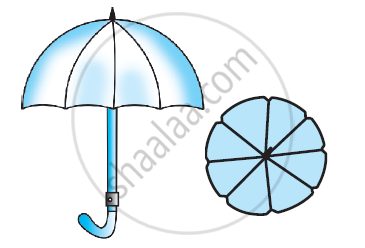Exercise 12.2 | Q 11 | Page 231

A car has two wipers which do not overlap. Each wiper has blade of length 25 cm sweeping through an angle of 115°. Find the total area cleaned at each sweep of the blades [Use Π  = 22/7]

Exercise 12.2 | Q 12 | Page 231

To warn ships for underwater rocks, a lighthouse spreads a red coloured light over a sector of angle 80° to a distance of 16.5 km. Find the area of the sea over which the ships warned. [Use π = 3.14]

Exercise 12.2 | Q 13 | Page 231

A round table cover has six equal designs as shown in figure. If the radius of the cover is 28 cm, find the cost of making the designs at the rate of Rs.0.35 per cm2. [Use sqrt3 = 1.7]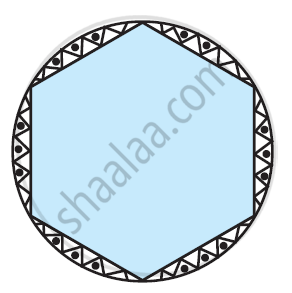Exercise 12.2 | Q 14 | Page 231

Tick the correct answer in the following:

Area of a sector of angle p (in degrees) of a circle with radius R is

• p/180  x 2ΠR

• p/180 x Π R2

• p/360 x 2Π R

• p/720 x 2Π R2

Exercise 12.3 [Pages 234 - 238]

### NCERT solutions for Class 10 Maths Chapter 12 Areas Related to Circles Exercise 12.3 [Pages 234 - 238]

Exercise 12.3 | Q 1 | Page 234

Find the area of the shaded region in the given figure, if PQ = 24 cm, PR = 7 cm and O is the centre of the circle. [Use Π = 22/7]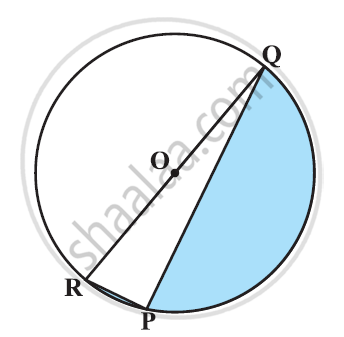Exercise 12.3 | Q 2 | Page 235

Find the area of the shaded region in the given figure, if radii of the two concentric circles with centre O are 7 cm and 14 cm respectively and ∠AOC = 40° [Use Π = 22/7]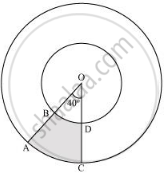Exercise 12.3 | Q 3 | Page 235

Find the area of the shaded region in the given figure, if ABCD is a square of side 14 cm and APD and BPC are semicircles.  [Use Π = 22/7]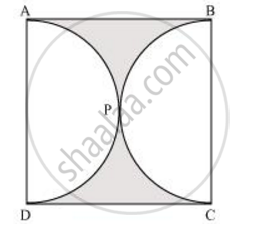Exercise 12.3 | Q 4 | Page 235

Find the area of the shaded region in the given figure, where a circular arc of radius 6 cm has been drawn with vertex O of an equilateral triangle OAB of side 12 cm as centre [Use Π = 22/7]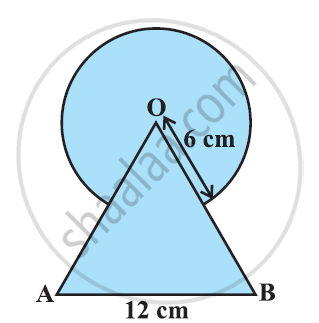Exercise 12.3 | Q 5 | Page 235

From each corner of a square of side 4 cm a quadrant of a circle of radius 1 cm is cut and also a circle of diameter 2 cm is cut as shown in the given figure. Find the area of the remaining portion of the square [Use Π = 22/7]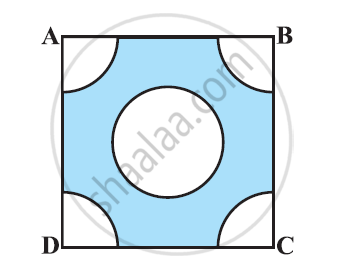Exercise 12.3 | Q 6 | Page 235

In a circular table cover of radius 32 cm, a design is formed leaving an equilateral triangle ABC in the middle as shown in the given figure. Find the area of the design (Shaded region). [Use Π = 22/7]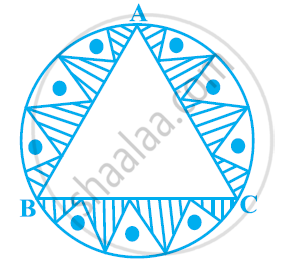Exercise 12.3 | Q 7 | Page 236

In the given figure, ABCD is a square of side 14 cm. With centres A, B, C and D, four circles are drawn such that each circle touches externally two of the remaining three circles. Find the area of the shaded region.  [Use Π = 22/7]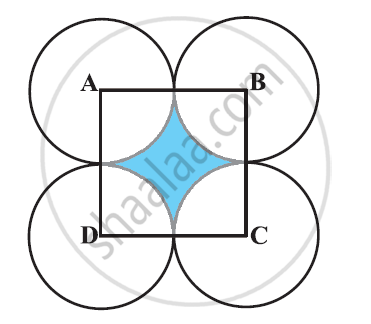Exercise 12.3 | Q 8 | Page 236

The given figure depicts a racing track whose left and right ends are semicircular.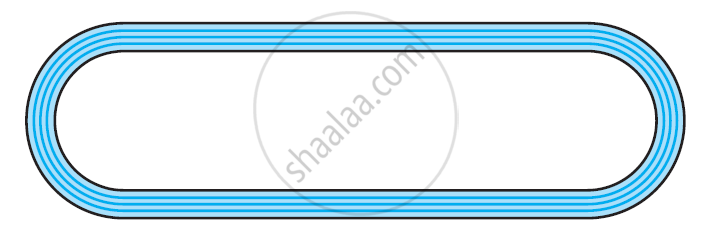The distance between the two inner parallel line segments is 60 m and they are each 106 m long. If the track is 10 m wide, find:

(i) The distance around the track along its inner edge

(ii) The area of the track

[Use Π = 22/7]

Exercise 12.3 | Q 9 | Page 236

In the given figure, AB and CD are two diameters of a circle (with centre O) perpendicular to each other and OD is the diameter of the smaller circle. If OA = 7 cm, find the area of the shaded region.  [Use Π = 22/7]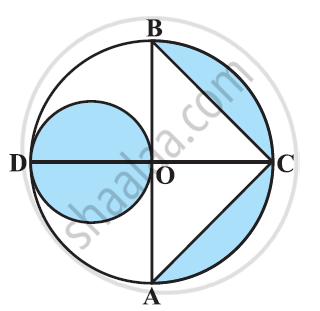Exercise 12.3 | Q 10 | Page 236

The area of an equilateral triangle ABC is 17320.5 cm2. With each vertex of the triangle as centre, a circle is drawn with radius equal to half the length of the side of the triangle (See the given figure). Find the area of shaded region. [Use π = 3.14 and sqrt3 = 1.73205]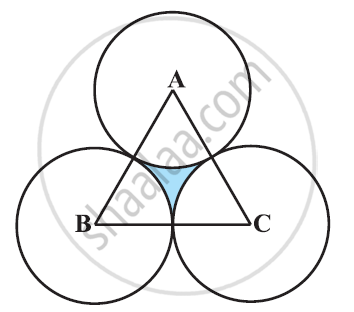Exercise 12.3 | Q 11 | Page 237

On a square handkerchief, nine circular designs each of radius 7 cm are made (see the given figure). Find the area of the remaining portion of the handkerchief.[Use Π = 22/7]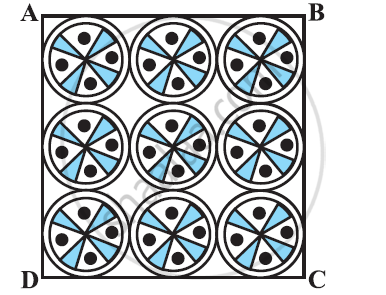Exercise 12.3 | Q 12 | Page 237

In the given figure, OACB is a quadrant of circle with centre O and radius 3.5 cm. If OD = 2 cm, find the area of the

[Use Π = 22/7]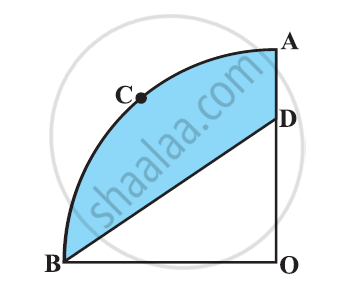Exercise 12.3 | Q 13 | Page 237

In fig. 3, a square OABC is inscribed in a quadrant OPBQ of a circle. If OA = 20 cm, find the area of the shaded region. (Use π = 3.14)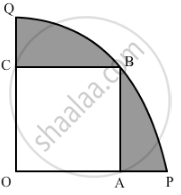Exercise 12.3 | Q 14 | Page 237

AB and CD are respectively arcs of two concentric circles of radii 21 cm and 7 cm and centre O (see the given figure). If ∠AOB = 30°, find the area of the shaded region. [Use Π = 22/7]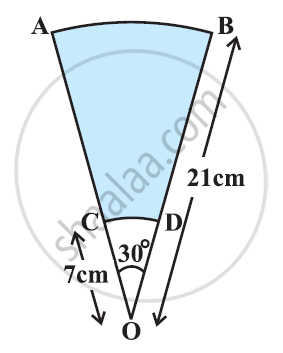Exercise 12.3 | Q 15 | Page 237

In the given figure, ABC is a quadrant of a circle of radius 14 cm and a semicircle is drawn with BC as diameter. Find the area of the shaded region. [Use Π = 22/7]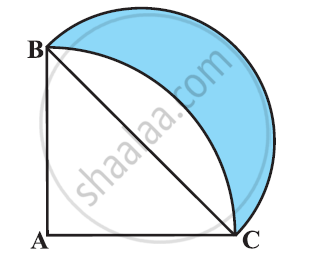Exercise 12.3 | Q 16 | Page 238

Calculate the area of the designed region in the given figure common between the two quadrants of circles of radius 8 cm each. [Use Π = 22/7]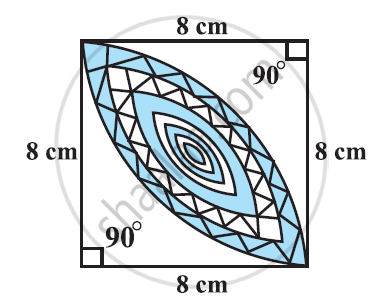## Solutions for Chapter 12: Areas Related to Circles

Exercise 12.1Exercise 12.2Exercise 12.3## NCERT solutions for Class 10 Maths chapter 12 - Areas Related to Circles

Shaalaa.com has the CBSE Mathematics Class 10 Maths CBSE solutions in a manner that help students grasp basic concepts better and faster. The detailed, step-by-step solutions will help you understand the concepts better and clarify any confusion. NCERT solutions for Mathematics Class 10 Maths CBSE 12 (Areas Related to Circles) include all questions with answers and detailed explanations. This will clear students' doubts about questions and improve their application skills while preparing for board exams.

Further, we at Shaalaa.com provide such solutions so students can prepare for written exams. NCERT textbook solutions can be a core help for self-study and provide excellent self-help guidance for students.

Concepts covered in Class 10 Maths chapter 12 Areas Related to Circles are Areas of Combinations of Plane Figures, Circumference of a Circle, Perimeter and Area of a Circle - A Review, Areas of Sector and Segment of a Circle, Area of Circle, Areas of Combinations of Plane Figures, Circumference of a Circle, Perimeter and Area of a Circle - A Review, Areas of Sector and Segment of a Circle, Area of Circle.

Using NCERT Class 10 Maths solutions Areas Related to Circles exercise by students is an easy way to prepare for the exams, as they involve solutions arranged chapter-wise and also page-wise. The questions involved in NCERT Solutions are essential questions that can be asked in the final exam. Maximum CBSE Class 10 Maths students prefer NCERT Textbook Solutions to score more in exams.

Get the free view of Chapter 12, Areas Related to Circles Class 10 Maths additional questions for Mathematics Class 10 Maths CBSE, and you can use Shaalaa.com to keep it handy for your exam preparation.